# AQA A Level Maths: Statistics复习笔记4.3.2 Normal Distribution - Calculations

###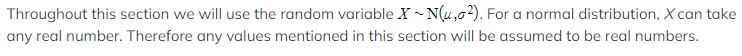Calculating Normal Probabilities

#### How do I find probabilities using a normal distribution?

•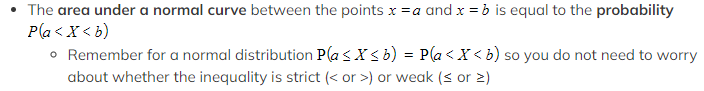The equation of a normal distribution curve is complicated so the area must be calculated numerically
• You will be expected to use distribution functions on your calculator to find the probabilities when working with a normal distribution

#### How do I calculate, P(X = x) ,the probability of a single value for a normal distribution?

• The probability of a single value is always zero for a normal distribution
• You can picture this as the area of a single line is zero
• P(X = x ) = 0
• Your calculator is likely to have a "Normal Probability Density" function
• This is sometimes shortened to NPD, Normal PD or Normal Pdf
• IGNORE THIS FUNCTION for this course!
• This calculates the probability density function at a point NOT the probability

#### How do I calculate, P(a < X < b)  the probability of a range of values for a normal distribution?

• You need a calculator that can calculate cumulative normal probabilities
• You want to use the "Normal Cumulative Distribution" function
• This is sometimes shortened to NCD, Normal CD or Normal Cdf
• You will need to enter:
• The 'lower bound' - this is the value a
• The 'upper bound' - this is the value b
• The 'µ' value - this is the mean
•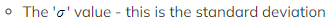Check the order carefully as some calculators ask for standard deviation before mean
• Remember it is the standard deviation (so if you have the variance then square root it)
• Always sketch a quick diagram to visualise which area you are looking for

#### How do I calculate, P(X>a) or P(X<b) for a normal distribution?

• You will still use the "Normal Cumulative Distribution" function
• P(X > a) can be estimated using an upper bound that is sufficiently bigger than the mean
• Using a value that is more than 4 standard deviations bigger than the mean is quite accurate
• Or an easier option is just to input lots of 9's for the upper bound (99999999.. or 1099)
• Similarly P(X < b) can be estimated using a lower bound that is sufficiently smaller than the mean
• Using a value that is more than 4 standard deviations smaller than the mean is quite accurate
• Or an easier option is just to input lots of 9's for the lower bound with a negative sign (-99999999... or -1099)
• This works because the probability that X is more than 3 standard deviations bigger than the mean is less than 0.0015
• This is the same for being 3 standard deviations less than the mean
• This reduces to less than 0.000032 when using 4 standard deviations

Are there any useful identities?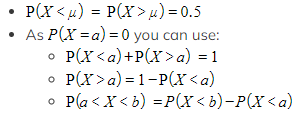These are useful when:

• The mean and/or standard deviation are unknown
• You only have a diagram
• You are working with the inverse distribution

#### Worked Example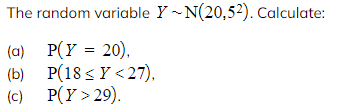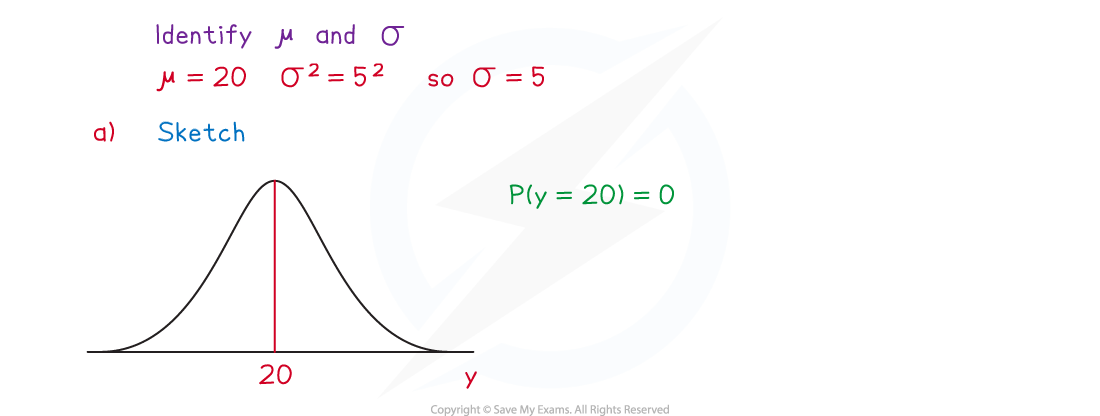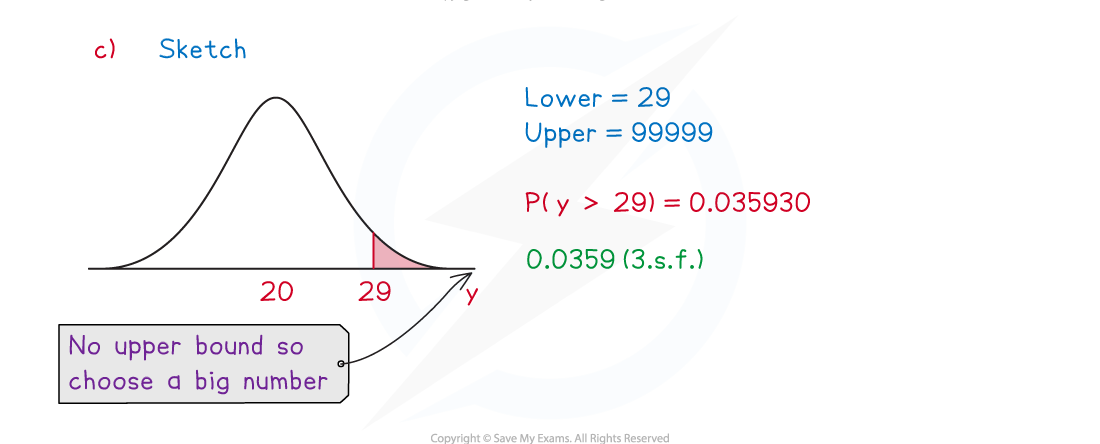### Inverse Normal Distribution

Given the value of P(X < a) how do I find the value of a ?

• Your calculator will have a function called "Inverse Normal Distribution"
• Some calculators call this InvN
• Given that P(X < a) = p  you will need to enter:
• The 'area' - this is the value p
• Some calculators might ask for the 'tail' - this is the left tail as you know the area to the left of a
• The 'μ' value - this is the mean
• The 'σ' value - this is the standard deviation
• Always check your answer makes sense
• If P(X < a)  is less than 0.5 then a should be smaller than the mean
• If P(X < a) is more than 0.5 then a should be bigger than the mean
• A sketch will help you see this

#### Given the value of P(X > a) how do I find the value of a  ?

• Given P(X > a) = p
• Use P(X < a) = 1 - P(X > a)  to rewrite this as P(X < a) = 1 - p
• Then use the method for (X < a) to find a
• If your calculator does have the tail option (left, right or centre) then you can use the "Inverse Normal Distribution" function straightaway by:
• Selecting 'right' for the tail
• Entering the area as 'p'

#### Worked Example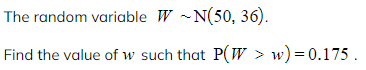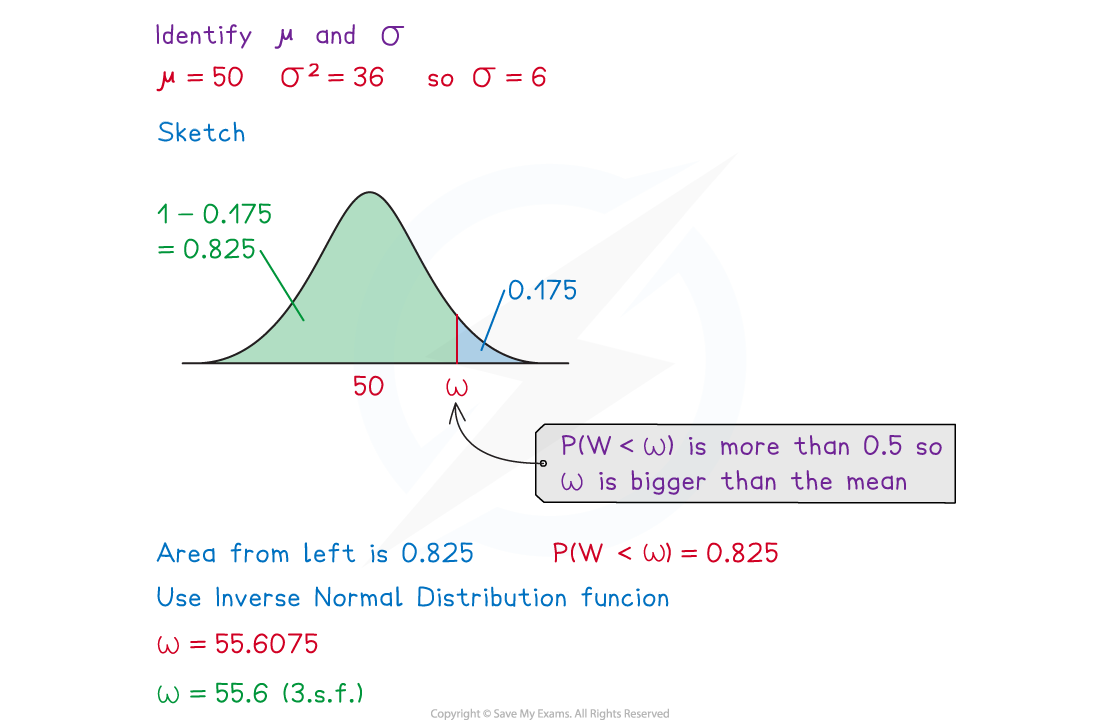#### Exam Tip

Always ask yourself two questions when using your calculator:

• Have you entered the mean and the standard deviation in the correct order?
• Have you entered the standard deviation correctly and not the variance?